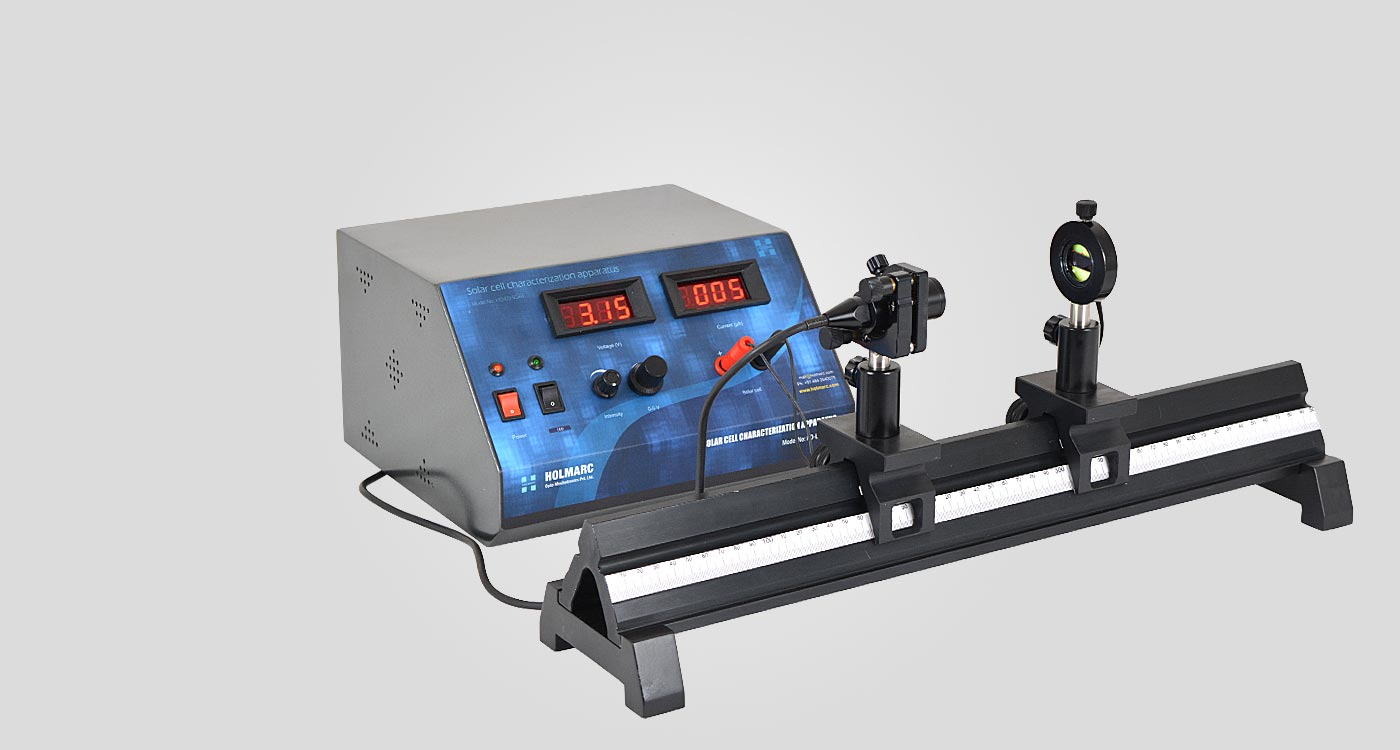Solar Cell Characterization

Apparatus

(I-V Characteristics)

Model: HO-ED-SC-01

Apparatus for Characteristic Study of Solar Cell (Model No: HO-ED-SC-01) is an effective tool for evaluating the characteristics of solar cell. This apparatus allows students in introductory physics course to plot I-V characteristics of a solar cell by a simple experiment. Important parameters such as fill factor, short circuit current, and open circuit voltage can be measured.

The apparatus consist of variable DC power supply, LED, solar cell and two multimeters (or an ammeter and a voltmeter). A variable DC power supply of 0-5V is used for onducting the experiment. Light source is provided by LED. The illumination intensity of the LED can be controlled. LED and solar cell is fixed to metallic casings for ease of placing in the experimental set up. The terminals of LED and solar cell are taken out through an insulated wire, which has a pair of alligator clips or a stereo connector.

Solar cells can absorb electromagnetic waves and convert the absorbed photon energy into electrical energy. It is a semiconductor device, which generates an emf when illuminated by light. When the energy of the incident photon is larger than the band gap, the photon can be absorbed by the semiconductor to create an electron-hole pair. The electrons and holes are then driven by the internal electric field in the diode to produce a photocurrent (light-generated current).

Experiment Examples

To plot the I - V characteristic curve of a solar cell

Set the solar cell facing towards a light source (LED) and bring the intensity of LED to the maximum using its rotary knob. Increase the voltage by changing the current through the solar cell and record current corresponding to the voltage. Plot the IV curve with current in the vertical axis, and voltage in the horizontal axis. This is the I-V characteristic curve of a solar cell.

To observe the relationship of current, voltage and power in a solar cell, and to identify the maximum power point, the short circuit current, and the open circuit voltage

Plot the IV curve with current in the vertical axis, and voltage in the horizontal axis. Note open circuit voltage (when current = 0), the short circuit current (when voltage = 0).

The current and voltage of each data set is then multiplied together giving a value for power. Plot another graph, with power in the vertical axis, and the voltage in the horizontal axis. This is the power graph. Indicate the maximum power in the power curve, and find the respective voltage and current.

To evaluate fill factor of the solar cell

The filling factor (FF) is defined to be Pm / (Isc.Voc), which represents an important parameter used to evaluate the quality of the solar cell.

Pm is the maximum output power of the solar cell, i.e., the maximum value of I * V .

Short-circuit current (Isc) is the output current of the solar cell when the external circuit is shorted, i.e., zero load resistance.

Open-circuit voltage (Voc) is the output voltage of the solar cell when the external circuit is open, i.e., infinite load resistance. Voc is also referred to as photovoltaic voltage.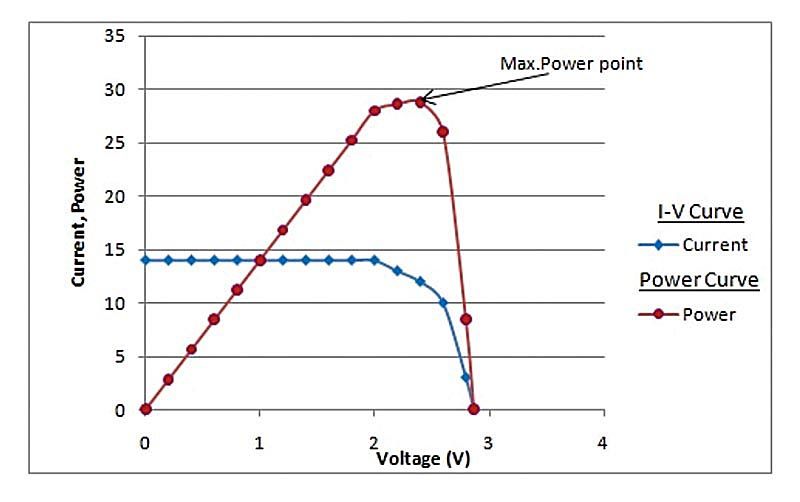Related Topics

Diode

Energy-band

Conduction band

Photocurrent

Valence band

Scope of Supply

Solar Cell Characterization control unit

Model No: ED-SC-01-SCCU
Voltage
:
0 - 5V
Input
:
230 V AC / 50 Hz
Quantity
:
1 no.Optical Rail

Model No: ED-SC-01-OR
Length
:
500mm
Material
:
Black anodized Aluminum alloy
Quantity
:
1 no.Kinematic LED mount

Model No: ED-SC-01-KLM
Material
:
Black anodized Aluminum alloy
:
:
+/-4 degrees
Quantity
:
1 nos.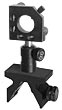Cell Mount

Model No: ED-SC-01-CM
Material
:
Black anodized Aluminum alloy
Diameter
:
30 mm
Quantity
:
1no. each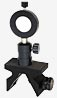LED module

Model No: ED-SC-01-LED
LED power
:
1 W
Color
:
White
Quantity
:
1no.Solar cell

Model No: ED-SC-01-SC
Quantity
:
1no.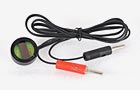AC Power code

Model No: ED-SC-01-APC
Type
:
3 core 3 Pin
Quantity
:
1no.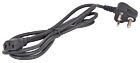|